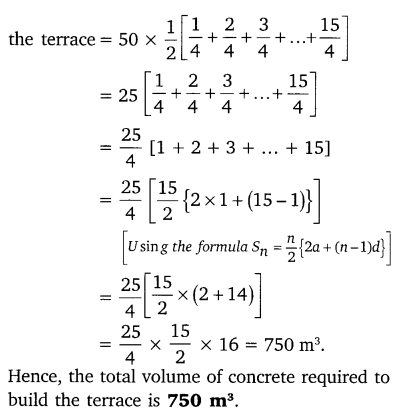Ex 5.4 Class 10 Question 1.
Which term of the AP: 121, 117. 113, ….., is its first negative term?
Solution:Ex 5.4 Class 10 NCERT Solutions Question 2.
The sum of the third term and the seventh term of an AP is 6 and their product is 8. Fibd the sum of first sixteen terms of the AP?
Solution: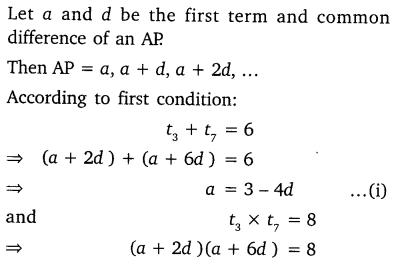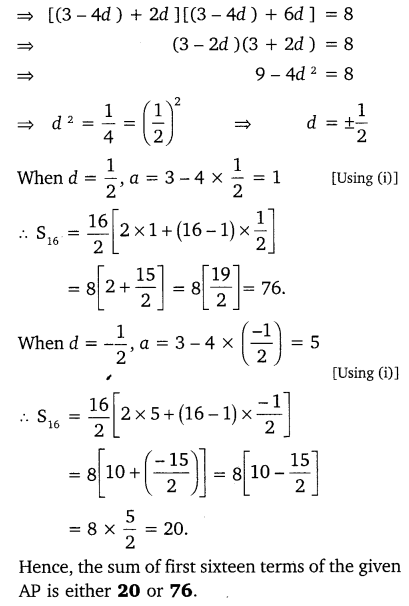Class 10 Ex 5.4 Question 3.
A ladder has rungs 25 cm apart. The rungs decrease uniformly in length from 45 cm at the bottom to 25 at the top. If the top and the bottom rungs are 212m apart, what is the length of the wood required for the rungs?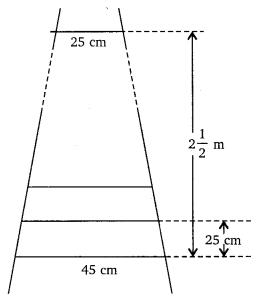Solution:Ex 5.4 Class 10 Question 4.
The houses of a row are numbered consecutively from 1 to 49. Show that there is value of x such that the sum of the numbers of the houses preceding the house numbered x is equal to the sum of the numbers of the houses following it. Find this value of x.
Solution: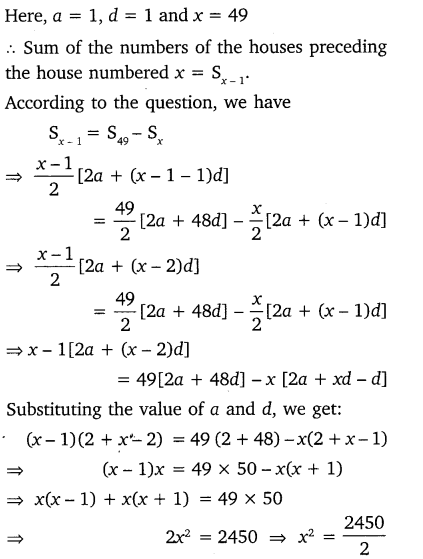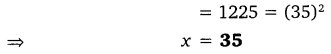Ex 5.4 Class 10 Question 5.
A small terrace at a football ground comprises of 15 steps each of which is 50  m long and built of solid concrete. Each step has a rise of 12m and a tread of 12m. Calculate the total volume of concrete required to build the terrace.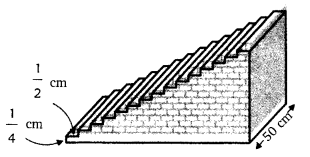Solution: